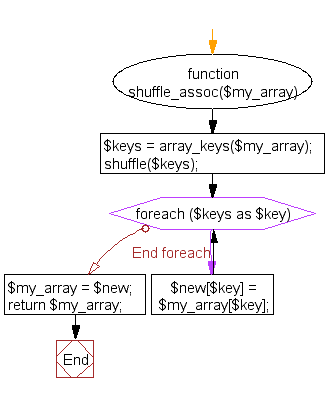﻿ PHP Array Exercise: Shuffle an associative array, preserving key, value pairs - w3resource# PHP Array Exercises : Shuffle an associative array, preserving key, value pairs

## PHP Array: Exercise-26 with Solution

Write a PHP function to shuffle an associative array, preserving key, value pairs.

Sample Solution:

PHP Code:

``````<?php
function shuffle_assoc(\$my_array)
{
\$keys = array_keys(\$my_array);

shuffle(\$keys);

foreach(\$keys as \$key) {
\$new[\$key] = \$my_array[\$key];
}

\$my_array = \$new;

return \$my_array;
}

\$colors = array("color1"=>"Red", "color2"=>"Green", "color3"=>"Yellow");

print_r(shuffle_assoc(\$colors));
?>
```
```

Sample Output:

```Array
(
[color1] => Red
[color2] => Green
[color3] => Yellow
)
```

Flowchart:PHP Code Editor:

What is the difficulty level of this exercise?

﻿

## PHP: Tips of the Day

Convert a PHP object to an associative array

Just typecast it

`\$array = (array) \$yourObject;`

From Arrays:

If an object is converted to an array, the result is an array whose elements are the object's properties. The keys are the member variable names, with a few notable exceptions: integer properties are unaccessible; private variables have the class name prepended to the variable name; protected variables have a '*' prepended to the variable name.These prepended values have null bytes on either side.

Example: Simple Object

```\$object = new StdClass;
\$object->foo = 1;
\$object->bar = 2;

var_dump( (array) \$object );
```

Output:

```array(2) {
'foo' => int(1)
'bar' => int(2)
}
```

Example: Complex Object

```class Foo
{
private \$foo;
protected \$bar;
public \$baz;

public function __construct()
{
\$this->foo = 1;
\$this->bar = 2;
\$this->baz = new StdClass;
}
}

var_dump( (array) new Foo );
```

Output (with \0s edited in for clarity):

```array(3) {
'\0Foo\0foo' => int(1)
'\0*\0bar' => int(2)
'baz' => class stdClass#2 (0) {}
}
```

Output with var_export instead of var_dump:

```array (
'' . "\0" . 'Foo' . "\0" . 'foo' => 1,
'' . "\0" . '*' . "\0" . 'bar' => 2,
'baz' =>
stdClass::__set_state(array(
)),
)
```

Typecasting this way will not do deep casting of the object graph and you need to apply the null bytes (as explained in the manual quote) to access any non-public attributes. So this works best when casting StdClass objects or objects with only public properties. For quick and dirty (what you asked for) it's fine.

Also see this in-depth blog post:

• Fast PHP Object to Array conversion

Ref : https://bit.ly/3fqiV2E